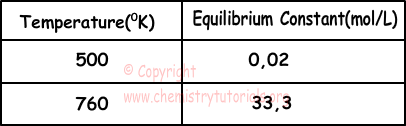## Chemical Equilibrium Exam1 and Problem Solutions

Chemical Equilibrium Exam1 and Problem Solutions

1. Following reaction is in equilibrium;

X(g) + 2Y(g) ↔ Z(g)  ∆H<0

If we increase temperature and pressure and add catalysts to this system, which ones of the following changes are true?

I. Rate of reaction increases

II. Equilibrium constant increases

III. Activation energy decreases

IV. Rate of reaction decreases

Solution:

X(g) + 2Y(g) ↔ Z(g)  ∆H<0

Using catalysts decrease activation energy and increase reaction rate. I and III true. Increasing temperature increases reaction rate whether it is endothermic or not.

Increasing pressure decrease volume and increase molar concentrations of matters. In exothermic reactions, increasing temperature decreases equilibrium constant.  II and IV are false.

2. Which ones of the followings can have "-" value in reactions?

I. Rate constant

II. Activation energy

III. Equilibrium constant

IV. Enthalpy of reaction

Solution:

Only enthalpy of reaction can have "-" value. Rate constant, activation energy, equilibrium constant are always positive.

3. Reaction given below is in equilibrium.

3O2(g) + 68kcal ↔ 2O3(g)

Which ones of the following applications increase O3 production?

I. Decreasing temperature and pressure

II. Increasing temperature and pressure

III. Increasing pressure, decreasing temperature

IV. Decreasing temperature, increasing amount of O2

Solution:

3O2(g) + 68kcal ↔ 2O3(g)

When we increase pressure and temperature, equilibrium point shifts to the products. II increases O3 production.

4. Following reaction includes matters in solid and gas phases.

A +2B ↔ C + D

Equilibrium constant equation of this reaction is;

Kc=[C]/[B]2

Which ones of the following statements are true for this reaction?

I. A and D are solids

II. Increasing pressure shifts equilibrium to the right

III. Adding A increases D production

Solution:

Matters in gas and aqueous are written to the equilibrium equation. Thus, A and D are solids.

A(s) +2B(g) ↔ C(g) + D(s)

Increasing temperature shift equation to the right.

Since A and D are solids, they do not affect equilibrium.

I and II are true, III is false.

5. XY5(g) ↔ XY3(g) + Y2(g)

This reaction has following equilibrium constants in given temperatures.According to these values; which ones of the following statements are true?

I. Reaction is exothermic

II. ∆H<0

III. Increasing temperature shifts equilibrium point to the right

Solution:

Reaction is endothermic. I is false.

Since reaction is endothermic; ∆H>0, II is false.

In endothermic reactions, increasing temperature increases value of equilibrium constant, however, in exothermic reactions increasing temperature decreases value of equilibrium constant. III is true.

Related